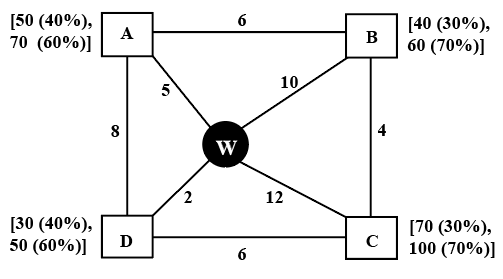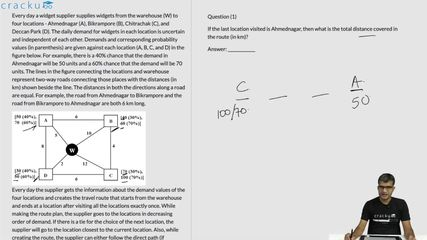### CAT 2022 Question Paper (Slot 2) - LRDI Question 10

Instructions

Every day a widget supplier supplies widgets from the warehouse (W) to four locations - Ahmednagar (A), Bikrampore (B), Chitrachak (C), and Deccan Park (D). The daily demand for widgets in each location is uncertain and independent of each other. Demands and corresponding probability values (in parenthesis) are given against each location (A, B, C, and D) in the figure below. For example, there is a 40% chance that the demand in Ahmednagar will be 50 units and a 60% chance that the demand will be 70 units. The lines in the figure connecting the locations and warehouse represent two-way roads connecting those places with the distances (in km) shown beside the line. The distances in both the directions along a road are equal. For example, the road from Ahmednagar to Bikrampore and the road from Bikrampore to Ahmednagar are both 6 km long.Every day the supplier gets the information about the demand values of the four locations and creates the travel route that starts from the warehouse and ends at a location after visiting all the locations exactly once. While making the route plan, the supplier goes to the locations in decreasing order of demand. If there is a tie for the choice of the next location, the supplier will go to the location closest to the current location. Also, while creating the route, the supplier can either follow the direct path (if available) from one location to another or can take the path via the warehouse. If both paths are available (direct and via warehouse), the supplier will choose the path with minimum distance.

Question 10

# If Ahmednagar is not the first location to be visited in a route and the total route distance is 29 km, then which of the following is a possible number of widgets delivered on that day?

Solution

Points to be noted:
1. Starts from the warehouse and ends at a location after visiting all the locations exactly once.
2. While making the route plan, the supplier goes to the locations in decreasing order of demand. If equal demand, goes to the nearest ones first.
3. While creating the route, the supplier can either follow the direct path (if available) from one location to another or can take the path via the warehouse(Prefers minimum distance).
Demand in all other locations should be less than or equal to the demand in first location. In the question, it is given that A is not first location. B and D cannot be first location. This implies C should be the first location.
It is given, total route distance is 29 km.
Warehouse to C is  12 km.
This implies remaining distance should be 29-12, i.e. 17km
This is only possible when C visits B, A and D later(4 + 6 + 7).
The order should be C - B - A - D
C's demand can be 70/100, B's demand should be 60, A's demand should be 50 and D's demand can be 30/50.
The possible number of widgets delivered can be
70 + 60 + 50 + 30 = 210
70 + 60 + 50 + 50 = 230
100 + 60 + 50 + 30 = 240
100 + 60 + 50 + 50 = 260